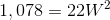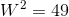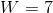# Intermediate Geometry : How to find the length of an edge of a prism

## Example Questions

### Example Question #1 : How To Find The Length Of An Edge Of A Prism

The volume of a prism is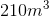.

Given the length is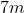and the height is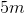, find the width of the prism.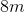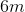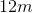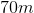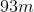Explanation:

The volume of a prism is length times width times height.

We are given the length is 7m and the width is 5m.

So, we plug these into our formula: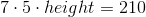.

We then solve for height: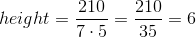.

The height is therefore 6m.

### Example Question #2 : How To Find The Length Of An Edge Of A Prism

Find the missing edge of the prism when its volume is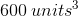.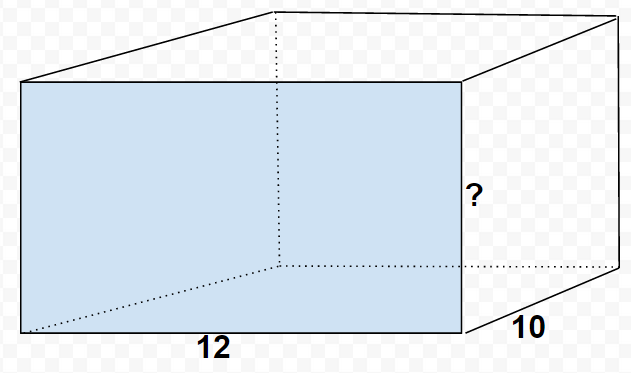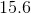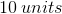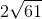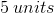Explanation:

The goal is to find the height of the rectangular prism with the given information of its width and length. The volume of a rectangular prism is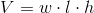, where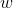is width andis height.

Because we're given the final volume and two of the three variables, we can substitute in the information we know and solve for the missing variable.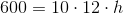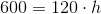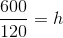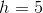Therefore, the height of the prism is.

### Example Question #3 : How To Find The Length Of An Edge Of A Prism

A rectangular prism has a volume of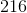cubic meters. Its length is twice its width, and its height is twice its length. What is the length of the prism?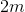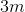Explanation:

If we letbe the width of our prism, then since the length is twice the width, our length would be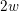.  Since the height is twice the length, our height would be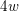.

Since the volume of a rectangular prism is simply the product of width, length, and height, we get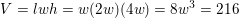We then simply solve for the width.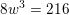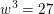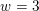Therefore, our width is 3.  Since our length is twice the width, the length is 6.

### Example Question #4 : How To Find The Length Of An Edge Of A Prism

A right, rectangular prism has a volume of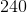cubic inches. Its width isinches and its height isinches. What is its length?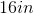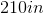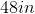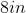Explanation:

The formula for the volume of a right, rectangular prism is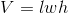, so substitute the known values and solve for lenght.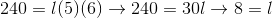.  So the length is 8 inches.

### Example Question #5 : How To Find The Length Of An Edge Of A Prism

The surface area of a right rectangular prism is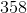square cm. Its length iscm and its height iscm. Find the width of the prism.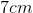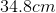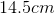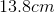Explanation:

Use the formula for finding the surface area of a right, rectangular prism,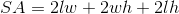and substitute the known values, and then solve for w.

So,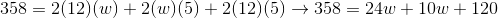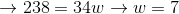So, the width is 7 cm.

### Example Question #6 : How To Find The Length Of An Edge Of A Prism

The height of a retangular prism is three times its width; its length is twice its width. Its surface area is 1,078 square inches. Give the width of the box.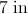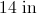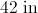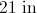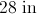Explanation:

Since the length of the box is twice the width and the height is three times the width,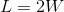and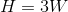The surface area of the box is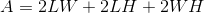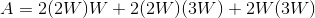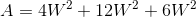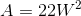To find the width: